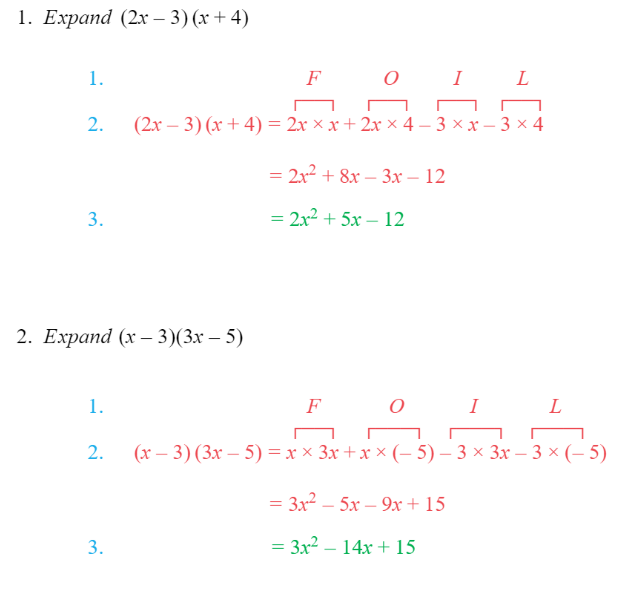# Edexcel IGCSE Maths 复习笔记 2.3.1 Expanding Two Brackets - Basics

Edexcel IGCSE Maths 复习笔记 2.3.1 Expanding Two Brackets - Basics

#### What is expanding two brackets?

• When we expand brackets in algebra we ‘get rid of’ the brackets by multiplying out
• When we expand two brackets, each term in one bracket gets multiplied by each term in the other bracket

#### Beware of minus signs

“ − × − = + ”“ − × + = − ”

#### How to multiply out two brackets

• Write FOIL above your working spaceF – First term in each bracket

O – Outer pair of termsI – Inner pair of terms

L – Last term in each bracket

• Write down the four multiplications
• Careful here – you have to be sure to include any minus signs!
• Simplify by collecting like terms (if there are any)

Worked Example